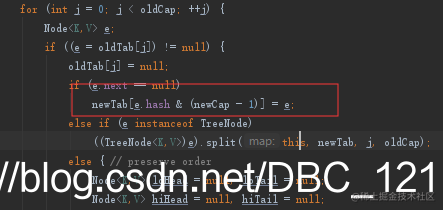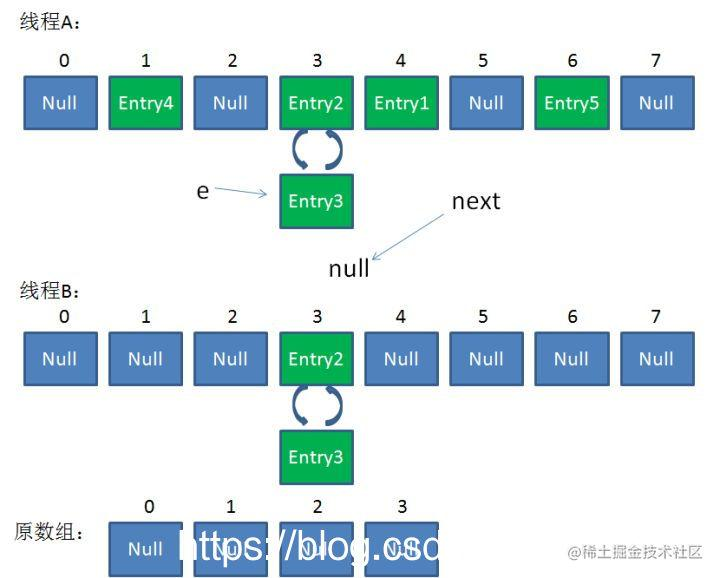•写在前面

•HashMap简介

•Hash函数

•初始容量和负载因子

•通过put和get看底层结构

•高并发下的HashMap

# •HashMap简介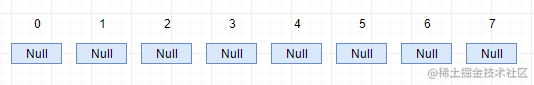# •Hash函数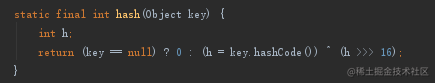``````index = HashCode(Key) & (Length - 1)

# •初始容量和负载因子

HashMap的实例又两个参数影响它的性能，分别是初始容量和复杂因子，在讲解前先说明，HashMap的默认初始容量是16，负载因子默认值是0.75（用于Hash计算），注意哦，初始容量设置太高或者负载因子设置太低，都会导致HashMap迭代性能下降。HashMap核心的东西是哈希表，初始容量和负载因子也是用于哈希表的，容量其实就是哈希表中桶的数量，初始容量只是哈希表在创建时的容量，负载因子是哈希表在其容量自动增加之前可以达到多满的一种尺度。当哈希表中的储存的数量超过了负载因子与当前容量的乘积时，就要对哈希表进行rehash操作（即重新构建内部的数据结构，相当于扩容，扩容的大小也有规定，无论是自动还是手动扩容，每次扩容之后的大小必须是2的幂）。这里我贴一下源码里的这两个东西。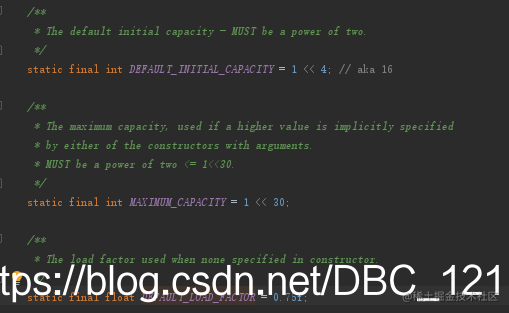# •通过put和get看底层结构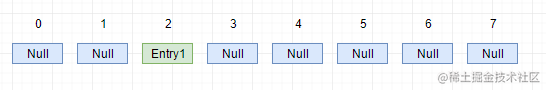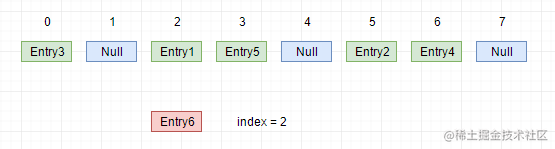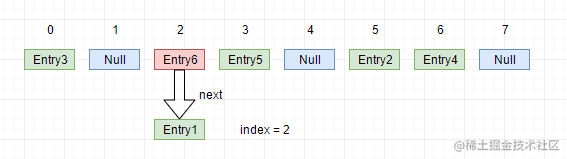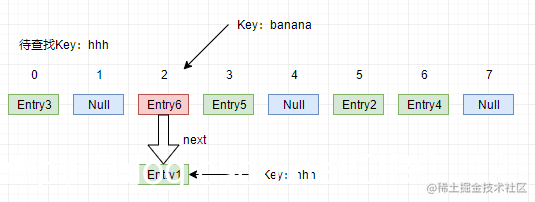# •高并发下的HashMap

``````    /**
* Initializes or doubles table size.  If null, allocates in
* accord with initial capacity target held in field threshold.
* Otherwise, because we are using power-of-two expansion, the
* elements from each bin must either stay at same index, or move
* with a power of two offset in the new table.
*
* @return the table
*/
final Node<K,V>[] resize() {
Node<K,V>[] oldTab = table;
int oldCap = (oldTab == null) ? 0 : oldTab.length;
int oldThr = threshold;
int newCap, newThr = 0;
if (oldCap > 0) {
if (oldCap >= MAXIMUM_CAPACITY) {
threshold = Integer.MAX_VALUE;
return oldTab;
}
else if ((newCap = oldCap << 1) < MAXIMUM_CAPACITY &&
oldCap >= DEFAULT_INITIAL_CAPACITY)
newThr = oldThr << 1; // double threshold
}
else if (oldThr > 0) // initial capacity was placed in threshold
newCap = oldThr;
else {               // zero initial threshold signifies using defaults
newCap = DEFAULT_INITIAL_CAPACITY;
}
if (newThr == 0) {
float ft = (float)newCap * loadFactor;
newThr = (newCap < MAXIMUM_CAPACITY && ft < (float)MAXIMUM_CAPACITY ?
(int)ft : Integer.MAX_VALUE);
}
threshold = newThr;
@SuppressWarnings({"rawtypes","unchecked"})
Node<K,V>[] newTab = (Node<K,V>[])new Node[newCap];
table = newTab;
if (oldTab != null) {
for (int j = 0; j < oldCap; ++j) {
Node<K,V> e;
if ((e = oldTab[j]) != null) {
oldTab[j] = null;
if (e.next == null)
newTab[e.hash & (newCap - 1)] = e;
else if (e instanceof TreeNode)
((TreeNode<K,V>)e).split(this, newTab, j, oldCap);
else { // preserve order
Node<K,V> loHead = null, loTail = null;
Node<K,V> hiHead = null, hiTail = null;
Node<K,V> next;
do {
next = e.next;
if ((e.hash & oldCap) == 0) {
if (loTail == null)
else
loTail.next = e;
loTail = e;
}
else {
if (hiTail == null)
else
hiTail.next = e;
hiTail = e;
}
} while ((e = next) != null);
if (loTail != null) {
loTail.next = null;
}
if (hiTail != null) {
hiTail.next = null;
}
}
}
}
}
return newTab;
}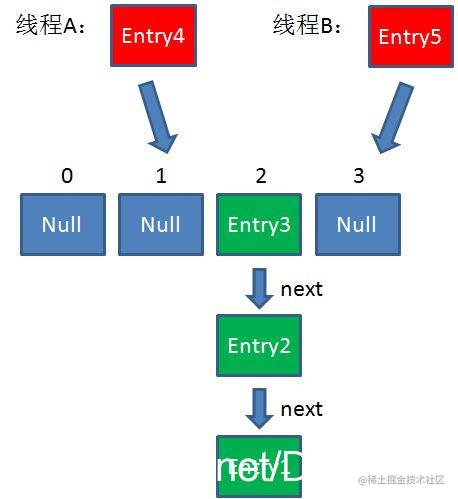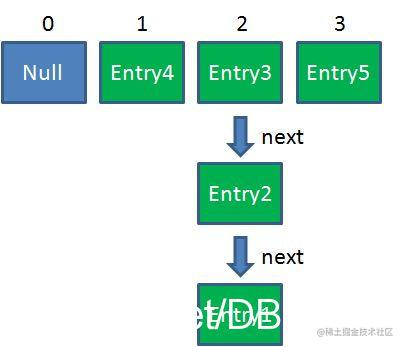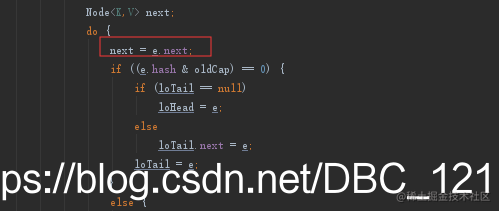``````e = Entry3
next = Entry2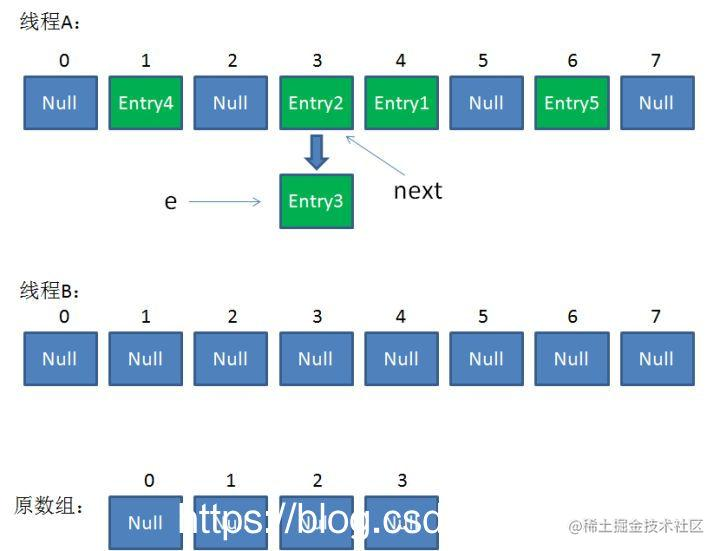``````e = Entry3
next = Entry2